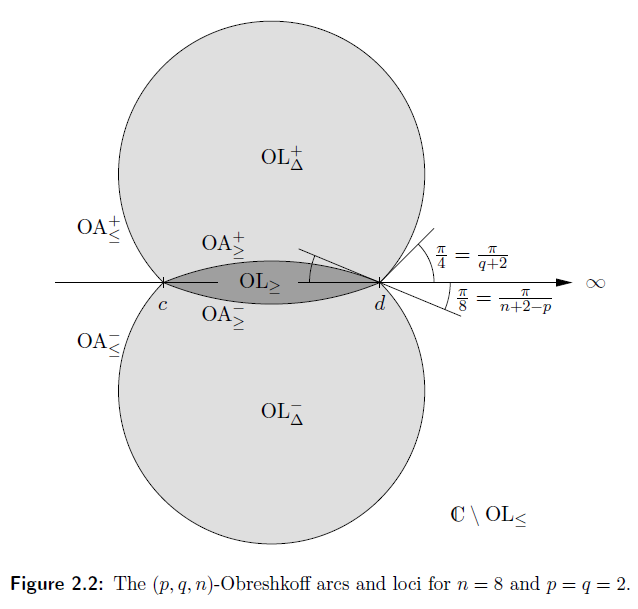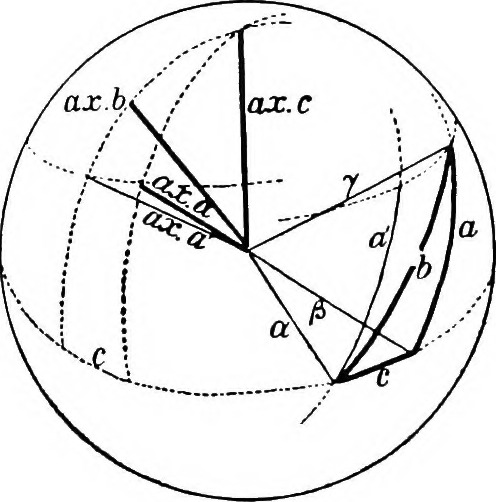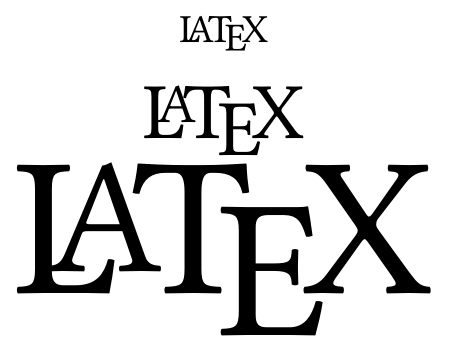## Learning about the world through critical thinking, data and programming.## Regular expressions, automata and monoids

In formal language theory the task is to specify, over some given alphabet, a set of valid strings. This is useful in searching for structures textual data through files (e.g. via grep), for specifying the syntactic structure of programming languages (e.g. in Bison or pandoc), and for generating output of a specified form (e.g. automatic computer science and mathematics paper generators).

An automoton is (roughly) a set of symbols, and a set of states, along with transitions for each state that take a symbol and return another state. They can be used to model (and verify) simple processes.

Automata can be brought into correspondence with formal languages in a very natural way; given an initial state s, and a sequence of symbols (a1, a2, …, an) the automata has a naturally assigned state (… ((s a1) a2) … an) (where “(state symbol)” represents the state obtained from the transition on symbol using state). Then if we nominate an initial state, and a set of “accepting” valid states, we say a string is in the language of the automata if and only if when applied to the initial state it ends in a final state.

This gives a very useful pairing in computer science; formal languages are useful tools, and automata (often) give an efficient way to implement them on a computer.## DVI by example

The Device Independent File Format (DVI) is the output format of Knuth’s TeX82; modern TeX engines (pdfTeX, luaTeX) output straight to Adobe’s Portable document format (PDF). However TeX82 and DVI still work as well today as they did when they were written; DVI files are easily cast to postscript or PDF.

The defining reference for DVI files is David R Fuch’s article in TUGboat Vol 3 No 2.

To find out what information is contained in a particular DVI file use Knuth’s dvitype, which outputs the operations contained in the bytecode in human readable format.

This article goes into gory detail the instructions contained in a very simple DVI file.## Algorithms for finding the real roots of polynomials

Given an degree n polynomial over the real numbers we are guaranteed there are at most n real roots by the fundamental theorem of algebra; but how do we find them? Here we explore the Vincent-Collins-Akritas algorithm.

It uses Descartes’ rule of signs: given a polynomial $$p(x) = a_n x^n + \cdots + a_1 x + a_0$$ the number of real positive roots (counting multiplicites) is bounded above by the number of sign variations in the sequence $$(a_n, \ldots, a_1, a_0)$$ .## Geometry and topology of division rings

Following from my last post (and Veblen and Young’s Projective Geometry) consider a projective plane satisfying the axioms:

1. Given two distinct points there is a unique line that both points lie on
2. Each line has at least three points which lie on it
3. Given a triangle any line that intersects two sides of the triangle intersects the third.
4. All points are spanned by d+1 points and no fewer.

Then for d>=3 is equivalent to the projective space of lines over a division ring (or skew field).

Kolmogorov asked the question what projective spaces can we do analysis on? In order to do things such as find tangent lines we are going to need some sort of topology.maths

## Geometry of division rings

It is fairly easy to construct a geometry from algebra: given a division ring K we form an n-dimensional vector space, the points being the elements of the field and a line being a translation of all (left) multiples of a non-zero vector, i.e. of the form $$\{a\mathbf{v} + \mathbf{c}| a \in K\}$$ for some fixed vectors $$\mathbf{v} \neq 0$$ and c.

Interestingly it’s just as possible to go the other way, if we’re careful about what we mean by a geometry. I will loosely follow Artin’s book Geometric Algebra. In particular we have the undefined terms of point, line and the undefined relation of lies on. Then, for a fixed positive integer, the axioms are:

1. Given two distinct points there is a unique line that both points lie on
2. Each line has at least three points which lie on it
3. Given a line and a point not on that line there exists a unique line lying on the plane containing them that the point lies on and no point of the first line lies on.
4. All points are spanned by d+1 points and no fewer.## LaTeXing Multiple Equations

In mathematics and the (hard) sciences it’s important to be able to write documents with lots of equations, lots of figures and lots of references efficiently. This can be done in, for example, Microsoft Word, but the mathematics and theoretical physics community heavily prefer $$\TeX$$ (and in particular $$\LaTeX$$ ), so the bottom line is if you want to get papers published you’re going to have to get good at it.

There are a lot of resources for learning $$\LaTeX$$ on the web, and a lot of people teach themselves from this (I know I did), but this can get you into some bad habits. For instance eqnarray gets the spacing around the equals signs all wrong. (I typeset my thesis using exclusively eqnarray and didn’t notice this until it was pointed out to me). So a lot of people advocate align from AMSTeX, but align has it’s limitations too; it only comes with one alignment tab &. If you want to make a comment at the end of multiple equations (like “for $$x \in X$$ “) or you want to have two equations and the second one breaks over two lines you can’t line the equations up properly; but there is a solution – IEEEeqnarray (which is an external class, IEEEtrantools, available from the IEEE). Stefan Moser has written an excellent paper covering everything I’ve said and much more, showing good ways to typeset equations.